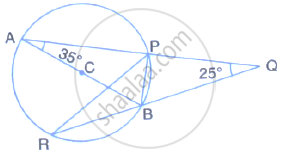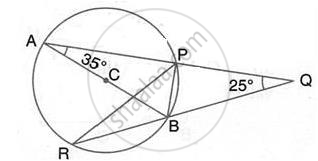Share

Ab is a Diameter of the Circle Apbr as Shown in the Figure. Apq and Rbq Are Straight Lines. Find : (I) ∠Prb, (Ii) ∠Pbr, (Iii) ∠Bpr. - ICSE Class 10 - Mathematics

ConceptArc and Chord Properties - Angles in the Same Segment of a Circle Are Equal (Without Proof)

Question

AB is a diameter of the circle APBR as shown in the figure. APQ and RBQ are straight lines.
Find : (i) ∠PRB,  (ii) ∠PBR,  (iii) ∠BPR.Solution(i)  ∠PRB = ∠PAB = 35°
(Angles subtended by the same chord on the circle are equal)

(ii) ∠BPA = 90°
(angle in a semicircle is a right angle)
∴ ∠BPQ = 90°
∴ ∠PBR = ∠BQP + ∠BPQ = 25° + 90° = 115°
(Exterior angle of a ∆ is equal to the sum of pair of interior opposite angles)

(iii) ABP = 90°- ∠BAP = 90° - 35° = 55°
∴ ∠ABR = ∠PBR = ∠ABP = 115° - 55° = 60°
∴ ∠APR = ABR = 60°

(Angles subtended by the same chord on the circle are equal)
∴ ∠BPR = 90° - ∠APR = 90° - 60° = 30°

Is there an error in this question or solution?
Solution Ab is a Diameter of the Circle Apbr as Shown in the Figure. Apq and Rbq Are Straight Lines. Find : (I) ∠Prb, (Ii) ∠Pbr, (Iii) ∠Bpr. Concept: Arc and Chord Properties - Angles in the Same Segment of a Circle Are Equal (Without Proof).
S